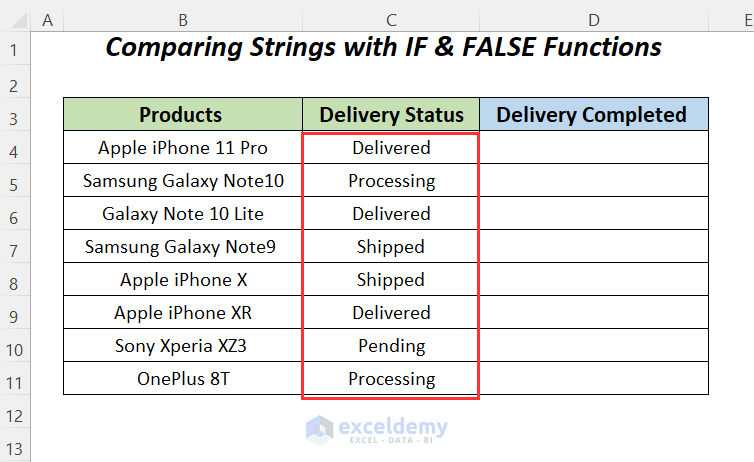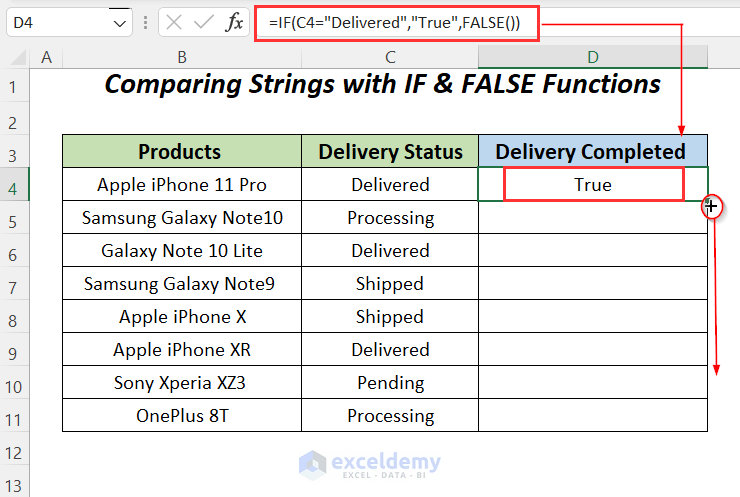# How to Use FALSE Function in Excel (7 Easy Examples)

Get FREE Advanced Excel Exercises with Solutions!

The FALSE function in Excel is one of the logical functions in Excel which gives FALSE as output. Using this function, we can declare the cell value as FALSE. This function is used widely in the IF function to print FALSE depending on various conditions. In this article, I will share the complete idea of how the FALSE function works in Excel independently and then with other Excel functions.

FALSE Function in Excel (Quick View)## Excel FALSE Function: Syntax & Arguments

⦿ Function Objective

The FALSE function gives us the word FALSE to make remarks or compare with others.

⦿ Syntax

`=FALSE()`As we can see from the picture above the FALSE function doesn’t take any argument in its parameter.

Note:

• Logical expressions will automatically generate TRUE and FALSE
• We can call the function “compatibility function” as we can use it for comparing with other worksheets.

⦿ Return Value

The FALSE function returns the logical value FALSE.

⦿ Version

The FALSE function has been introduced in Excel 2000 version and is available for all versions after that.

## 7 Examples to Use FALSE Function in Excel

Here, we have a dataset of some products with their last two years’ total sales. Using this dataset, we will explain most of the following examples to demonstrate the uses of the FALSE function.For making this article, we are using Microsoft Excel 365 version, you can use whatever you want.

### Example-1: Getting Output as FALSE by Using Only FALSE Function

Here, we have seen that none of the sales values in Columns C and D are greater than 500,000 and so we want to put down FALSE in column E using the FALSE function. This function will only give us the logical value FALSE.• Type the following function in cell E4.
`=FALSE()`As a result, we are getting FALSE as a result in column E.### Example-2: Multiplying, Dividing, Adding, and Subtracting Different Numbers with FALSE Function

Here, we will be adding, subtracting, multiplying, and dividing the values of column C with the FALSE function and will see the results. The FALSE function is similar to zero numerically.• Use the following formula to add the values with the FALSE function.
`=FALSE()+C4`

Here, we are getting results similar to the sales values in 2020 as the FALSE function is giving zero.• Secondly, we will try to subtract the FALSE function from the sales values using the following formula.
`=C4-FALSE()`

In this case, also, we are getting results similar to the sales values in 2020 as the FALSE function is giving zero.• But if you subtract the sales values from the function like the following
`=FALSE()-C11`

Then, you will get a negative value.• Thirdly, we will multiply the sales values with the FALSE function.
`=C4*FALSE()`

The resultant values will be zero as we are multiplying the sales values with 0.• Finally, we will divide the FALSE function by the sales values.
`=FALSE()/C4`

The resultant values will be zero as we are dividing 0 by the sales values.• But if you divide the sales values by the function like the following
`=C11/FALSE`

Then, you will get the #DIV/0! error.### Example-3: Using IF and FALSE Functions to Compare Two Numbers in Excel

Now we will compare the sales values between two years and find out if the sales of each product increased or not.• Type the formula in cell E4.
`=IF(D4>C4,"True",FALSE())`

Formula Explanation

• `Here inside ``the IF function``, ``D4>C4 ``is our logical condition.`
• `We are checking if the sales in`` column` `D`` are greater than the sales in ``column` `C`` or not. If the result is yes then we will print the ``“True”`` message otherwise ``the FALSE function`` will return`` FALSE``.`
• Drag down the Fill Handle.Finally, you will get True for the sales values which are increased from 2019 to 2020, and FALSE for the sales values which are not increased.Read More: How to Use TRUE Function in Excel (10 Ideal Examples)

### Example-4: Applying IF and FALSE Functions to Compare Strings

Now let’s say we have the same product list along with their delivery status. The status could be Delivered, Shipped, Processing, or Pending. Now we will find out the products which are already delivered.

###• Type the formula in cell D4 and copy it down up to D11
`=IF(C4="Delivered","True",FALSE())`

Formula Explanation

• `C4="Delivered" ``is the condition.`
• `"True" ``will be printed if the condition is true.`
• `The FALSE() function`` will return ``FALSE`` if the condition is false.`

###Finally, you will get True for the delivered condition, and FALSE for the shipped and processing conditions.### Example-5: Combining COUNTIF and FALSE Functions

Now for the previous example, we will count the number of incomplete deliveries. We need to count the cells where Delivery Completed is equal to FALSE and so we will use the COUNTIF function additionally. For more details about this function, you can check these links.
COUNTIF Multiple Ranges Same Criteria in Excel
COUNTIF between Two Cell Values in Excel

So, our scenario will be like this:• Enter the formula in cell C13.
`=COUNTIF(\$D\$4:\$D\$11, FALSE())`

Formula Explanation

• `\$D\$4:\$D\$11 ``this is the range from where we will find the`` FALSE``.`
• `FALSE () ``will be used for comparing values. From the range, we will compare cell values with this function and count the ``FALSE``.`### Example-6: Using IF, AND, SUM, and FALSE Functions to Determine Pass and Fail

Now let’s move to a complex example. Let’s assume we have a dataset of some students’ IDs, Names, and subject-wise marks. Let’s say the passing threshold value is 50. Now if any student gets less than 50 marks on any subject then it will be considered a failure and print FALSE as a message. Otherwise, it will show the average marks.• Enter the formula in cell H4 and copy it down to H12.
`=IF(AND(D4>=50, E4>=50, F4>=50, G4>=50), SUM(D4:G4)/4, FALSE() )`

Formula Explanation

• `Here, ``SUM(D4:G4)/4 ``is calculating the average marks for each student.` The SUM function `is for calculating the sum of each row and as we have ``4`` subject marks that’s why the summed value is divided by ``4``.`
• `AND(D4>=50, E4>=50, F4>=50, G4>=50) ``will use` the AND function `to check if there is any subject column that is less than ``50``. If any of the values is less than ``50``, then the whole return value will be ``FALSE``, otherwise, the average marks will be returned.`
• `Lastly, ``the FALSE() function`` is used to print ``FALSE`` if the condition is false.`Eventually, we will get the obtained marks for each student who has received marks greater than 50, the students who have not received this minimum mark will get FALSE here.### Example-7: Finding FALSE Values

In this section, we will see how to find out the FALSE value from any cell. For this, we will use the same dataset used in Example 3. Here we will not directly use the FALSE function. We will use the NOT function to find the FALSE value from cells.• Enter the formula in cell F4 and copy it down up to F11.
`=NOT(E4)`

Formula Explanation

The syntax of the NOT function is:

`NOT(logical)`
Logical —–> This is a required argument. The condition which you want to choose should be selected here.In this way, we are getting TRUE for the cells which contain FALSE and it is representing that the sales have decreased.## How to Use TRUE and FALSE Functions in Excel

We can use the combination of the TRUE and FALSE functions to do the same task as in Example 3.• Enter the formula in cell E4 and copy it down up to E11
`=IF(D4>C4,TRUE(),FALSE())`

Formula Explanation

• `Here inside ``the IF function``, ``D4>C4 ``is our logical condition.`
• `We are checking if the sales in`` column` `D`` are greater than the sales in ``column` `C`` or not. If the result is yes then ``the TRUE function`` will print the ``TRUE`` message otherwise ``the FALSE function`` will return`` FALSE``.`Finally, you will get TRUE for the sales values which are increased from 2019 to 2020, and FALSE for the sales values which are not increased.## Things to Remember

• The FALSE function always returns a logical FALSE
• FALSE & FALSE() both are similar.
• The function does not require any argument.
• It has a numeric value of 0.

## Conclusion

This is all about the FALSE function in Excel and its different applications. If you have any other method of utilizing this function, then please feel free to share it with us.

## Related ArticlesHello! Welcome to my Profile. Currently, I am working and researching Microsoft Excel, and here I will be posting articles related to this. My last educational degree is BSc, and my program was Computer Science and Engineering from American International University-Bangladesh. I am a Computer Science graduate with a great interest in research and development. Always try to gather knowledge from various sources and try to make innovative solutions.

We will be happy to hear your thoughtsAdvanced Excel Exercises with Solutions PDF